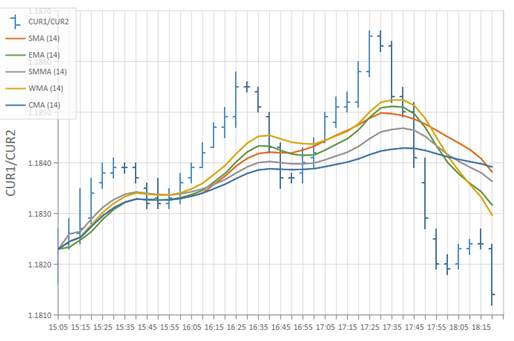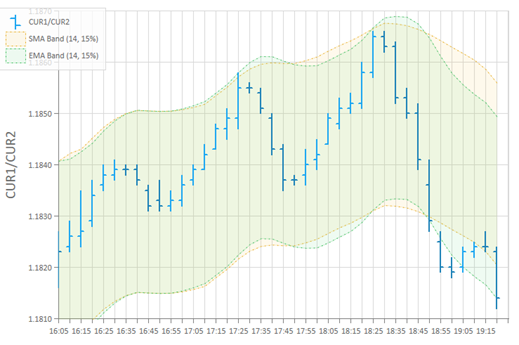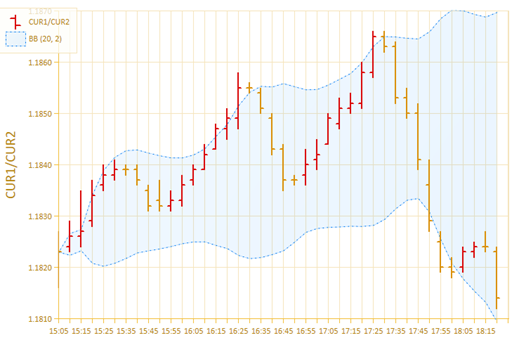# Stock Chart (Overlays and Envelopes)BCGControlBar Pro (MFC)BCGSuite (MFC)BCGControlBar for .NET

The following chart formulas are implementing stock charts with technical indicator and envelopes:

• The CBCGPChartMAFormula implements a formula that computes an output series as a moving average.
• The CBCGPChartMAENVFormula class implements the functionality of Moving Average Envelops (MAENV) technical indicator.
• The CBCGPChartBollingerBandsFormula class implements the functionality of Bollinger Bands (BB) technical indicator.

#### Moving average (MA):#### Moving average bands (MAENV):#### Bollinger band (BB):